### SAT数学：代数例题 11

2019-09-21 SAT-数学 阅读

# Rank: Medium Topic: Algebra, Solving an Equation with Radicals

Below is a question from a recent SAT exam. It is a non-calculator question that would typically take a student 1-2 minutes to complete.What are all values of x that satisfy the equation above?

I.1
II.9

A) I only
B) II only
C) I and II
D) Neither I nor II

To answer this question, we must first remove the square root. We do this by doing the opposite of square rooting, which is, of course, squaring.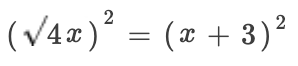Notice how the right-hand side is now in brackets as the terms are not squared individually.

So, the left-hand side just becomes 4x and the right-hand side we work out by expanding as a set of double brackets.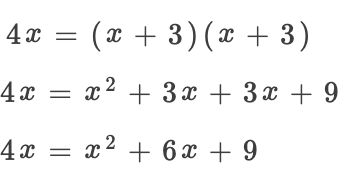We then re-arrange into the standard quadratic form.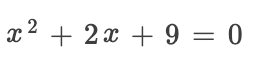This equation cannot be solved by factoring so we would typically use the quadratic formula. However, this equation does not have any real roots. We can test this with the formula: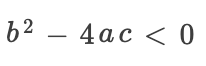So we use the values from our quadratic.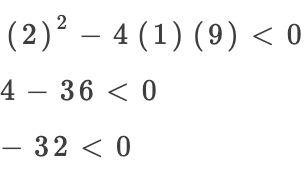Therefore, neither 1 or 9 satisfy the equation. So, the answer is D.• 地址：401-5811 Cooney Rd, Richmond, BC
• 地址：3030-8171 Ackroyd Rd, Richmond, BC
• 客服热线：604-370-0579 & 604-370-2569
• 联系邮箱：service@forbest.com

• 地址：江苏省无锡市滨湖区绣溪路50号K-PARK商务大楼3号楼3楼317室
• 客服热线：181-1861-8855

•官方公众号
•加拿大官方客服
•中国官方客服